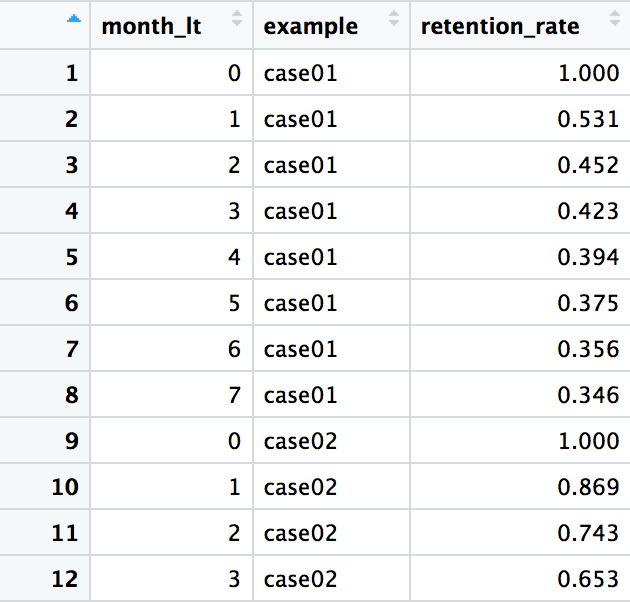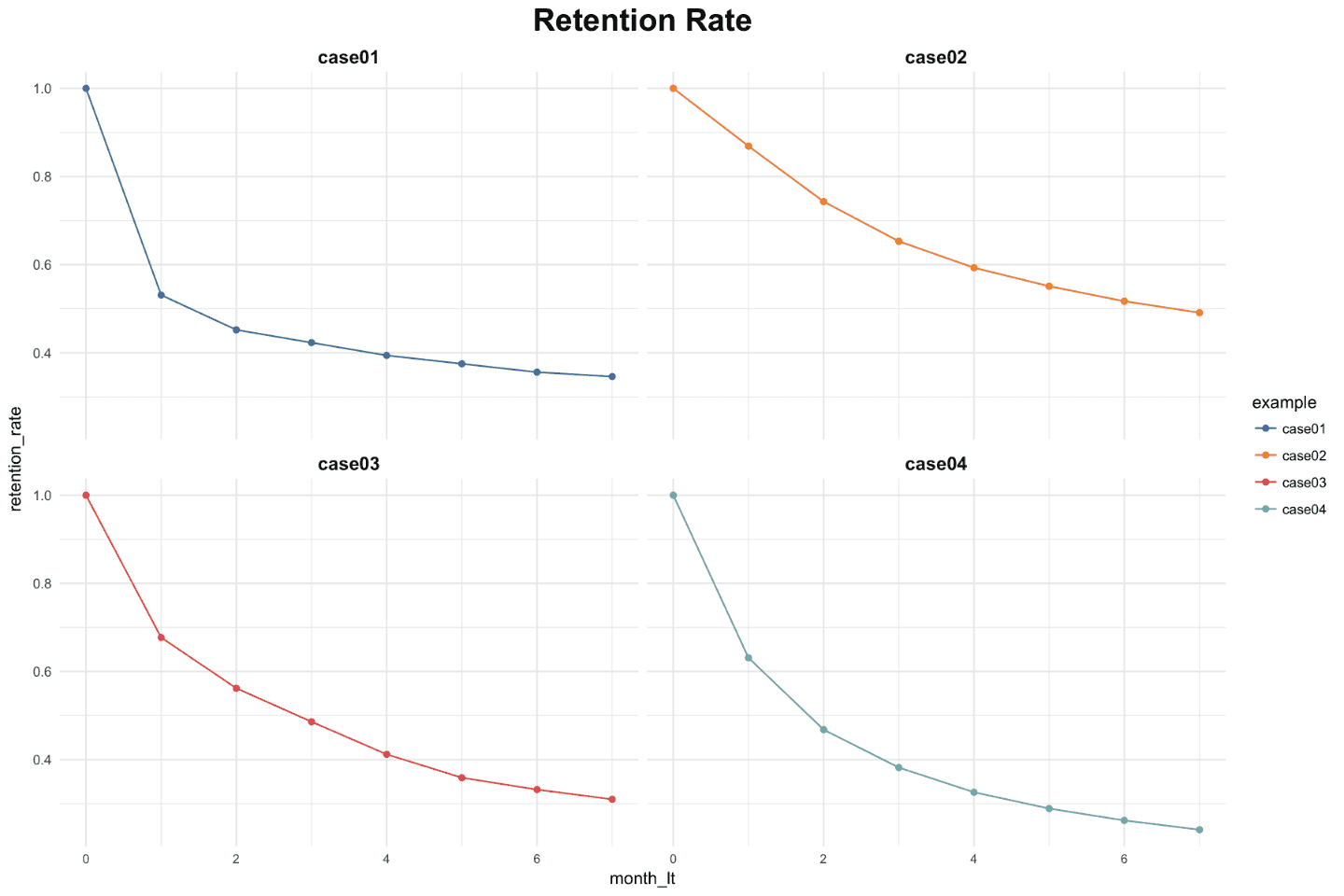[This article was first published on R language – AnalyzeCore by Sergey Bryl' – data is beautiful, data is a story, and kindly contributed to R-bloggers]. (You can report issue about the content on this page here)
Want to share your content on R-bloggers? click here if you have a blog, or here if you don't.

Customers lifetime value (LTV or CLV) is one of the cornerstones of product analytics because we need to make a lot of decisions for which the LTV is a necessary or at least very significant factor. In this article, we will focus on products/services/applications with recurring subscription paymentsCustomers lifetime value (LTV or CLV) is one of the cornerstones of product analytics because we need to make a lot of decisions for which the LTV is a necessary or at least very significant factor. In this article, we will focus on products/services/applications with recurring subscription payments.

Predicting LTV is a common issue for a new, recently launched product/service/application when we don’t have a lot of historical data but want to calculate LTV as soon as possible. Even though we may have a lot of historical data on customer payments for a product that is active for years, we can’t really trust earlier stats since the churn curve and LTV can differ significantly between new customers and the current ones due to a variety of reasons.

Therefore, regardless of whether our product is new or “old”, we attract new subscribers and want to estimate what revenue they will generate during their lifetimes for business decision-making.

This topic is closely connected to the Cohort Analysis and if you are not familiar with the concept, I recommend that you read about it and look at other articles I wrote earlier on this blog.

As usual, in the article, we will review an example of LTV projection using the R language although this approach can be implemented even in MS Excel.

Ok, let’s start. In order to predict the average LTV for the product/service/application with a constant subscription payment, it suffices to know how many subscribers will churn (or be retained) at the end of each subscription period. I’ve collected four examples: two from my practice and two from research that demonstrates the effectiveness of the approach. The above examples refer to different businesses and show both monthly and annual subscriptions, but for convenience, they are all monthly ones.

click to expand R code

```library(tidyverse)
library(reshape2)

# retention rate data
df_ret <- data.frame(month_lt = c(0:7),
case01 = c(1, .531, .452, .423, .394, .375, .356, .346),
case02 = c(1, .869, .743, .653, .593, .551, .517, .491),
case03 = c(1, .677, .562, .486, .412, .359, .332, .310),
case04 = c(1, .631, .468, .382, .326, .289, .262, .241)
) %>%
melt(., id.vars = c('month_lt'), variable.name = 'example', value.name = 'retention_rate')

ggplot(df_ret, aes(x = month_lt, y = retention_rate, group = example, color = example)) +
theme_minimal() +
facet_wrap(~ example) +
scale_color_manual(values = c('#4e79a7', '#f28e2b', '#e15759', '#76b7b2')) +
geom_line() +
geom_point() +
theme(plot.title = element_text(size = 20, face = 'bold', vjust = 2, hjust = 0.5),
axis.text.x = element_text(size = 8, hjust = 0.5, vjust = .5, face = 'plain'),
strip.text = element_text(face = 'bold', size = 12)) +
ggtitle('Retention Rate')
```

The initial data is as follows:month_lt – month (or subscription period) of customer’s lifetime,

examplethe name of the example,

retention_rate – the percentage of subscribers who have maintained the subscription.

Next, the visualization of four retention curves. Looks very familiar, doesn’t it?Let’s consider the approach for predicting LTV. As I noted earlier, when we deal with the constant subscription payment, we can predict average subscribers’ LTV by projecting a retention curve. In other words, we need to continue “drawing” the curve that starts with factual points we have for the future periods.

However, instead of using the traditional approach (i.e. a regression model), we can use an alternative probabilistic method that Peter Fader and Bruce Hardie suggested.

The authors demonstrated the concept which, despite having simplified assumptions, shows excellent results in practice. There are a lot of materials on the Internet about the method, so we won’t go into details, we will just highlight the core ideas.

We assume that each subscriber has a certain constant churn probability and after the end of each period, they “flip a coin to decide whether to churn or to maintain the subscription”, where the probability works. Since the probability of retaining subscribers in each period is calculated by multiplying the previous probabilities, the subscriber’s lifetime is characterized by the Shifted Geometric Distribution.

Despite the fact that the specific probability for each subscriber is unknown to us, we assume that it is characterized by the Beta Distribution, which fits the goal ideally given two facts. Firstly, it has an interval from 0 to 1 (as probability). Secondly, it is flexible. Namely, we can change (tune, in our case) the distribution form by changing two parameters alpha and beta.

By combining these two assumptions through a mathematical apparatus, the authors came to the idea that subscriber’ lifetimes can be characterized by the Shifted-Beta-Geometric (sBG) distribution (which in turn is determined by two parameters: alpha and beta). However, alpha and beta are unknown to us. We can compute them by maximizing the likelihood estimation that they fit the observed factual data of subscribers’ retention and, accordingly, keep our retention curve for the future periods.

Let’s check the accuracy of the predictions on the available data. We will assume that we only know the first month’s retention, then the first and the second months, and so on until we predict all subsequent periods until the end of the 7th month. I wrote a simple loop for this, which you can apply to each example:

click to expand R code

```# functions for sBG distribution
churnBG <- Vectorize(function(alpha, beta, period) {
t1 = alpha / (alpha + beta)
result = t1
if (period > 1) {
result = churnBG(alpha, beta, period - 1) * (beta + period - 2) / (alpha + beta + period - 1)
}
return(result)
}, vectorize.args = c("period"))

survivalBG <- Vectorize(function(alpha, beta, period) {
t1 = 1 - churnBG(alpha, beta, 1)
result = t1
if(period > 1){
result = survivalBG(alpha, beta, period - 1) - churnBG(alpha, beta, period)
}
return(result)
}, vectorize.args = c("period"))

MLL <- function(alphabeta) {
if(length(activeCust) != length(lostCust)) {
stop("Variables activeCust and lostCust have different lengths: ",
length(activeCust), " and ", length(lostCust), ".")
}
t = length(activeCust) # number of periods
alpha = alphabeta
beta = alphabeta
return(-as.numeric(
sum(lostCust * log(churnBG(alpha, beta, 1:t))) +
activeCust[t]*log(survivalBG(alpha, beta, t))
))
}

df_ret <- df_ret %>%
group_by(example) %>%
mutate(activeCust = 1000 * retention_rate,
lostCust = lag(activeCust) - activeCust,
lostCust = ifelse(is.na(lostCust), 0, lostCust)) %>%
ungroup()

ret_preds01 <- vector('list', 7)
for (i in c(1:7)) {

df_ret_filt <- df_ret %>%
filter(between(month_lt, 1, i) == TRUE & example == 'case01')

activeCust <- c(df_ret_filt\$activeCust)
lostCust <- c(df_ret_filt\$lostCust)

opt <- optim(c(1, 1), MLL)
retention_pred <- round(c(1, survivalBG(alpha = opt\$par, beta = opt\$par, c(1:7))), 3)

df_pred <- data.frame(month_lt = c(0:7),
example = 'case01',
fact_months = i,
retention_pred = retention_pred)
ret_preds01[[i]] <- df_pred
}

ret_preds01 <- as.data.frame(do.call('rbind', ret_preds01))
```

The visualization of the predicted retention curves and mean average percentage error (MAPE) is the following:

As you can see, in most cases, the accuracy of the predictions is very high even for the first month’s data only and it significantly improves when adding historical periods.

Now, in order to obtain the average LTV prediction, we need to multiply the retention rate by the subscription price and calculate the cumulative amount for the required period. Let’s suppose we want to calculate the average LTV for case03 based on two historical months with a forecast horizon of 24 months and a subscription price of \$1. We can do this using the following code:

click to expand R code

```### LTV prediction ###
df_ltv_03 <- df_ret %>%
filter(between(month_lt, 1, 2) == TRUE & example == 'case03')

activeCust <- c(df_ltv_03\$activeCust)
lostCust <- c(df_ltv_03\$lostCust)

opt <- optim(c(1, 1), MLL)
retention_pred <- round(c(survivalBG(alpha = opt\$par, beta = opt\$par, c(3:24))), 3)

df_pred <- data.frame(month_lt = c(3:24),
retention_pred = retention_pred)

df_ltv_03 <- df_ret %>%
filter(between(month_lt, 0, 2) == TRUE & example == 'case03') %>%
select(month_lt, retention_rate) %>%
bind_rows(., df_pred) %>%
mutate(retention_rate_calc = ifelse(is.na(retention_rate), retention_pred, retention_rate),
ltv_monthly = retention_rate_calc * 1,
ltv_cum = round(cumsum(ltv_monthly), 2))
```

We’ve obtained the average LTV of \$9.33. Note that we’ve used actual data for the observed periods (from 0 to 2nd months) and the predicted retention for the future periods (from 3rd to 24th months).

A couple of tips from the practice:

• In order to get a retention or LTV prediction as soon as possible, for example, for a monthly subscription, you can create weekly/decadal (or even daily, if the number of subscribers is large enough) cohorts. Therefore, you can obtain the prediction after the month and the week (or decade or day) period instead of a two-month one.
• In order to improve the accuracy of predictions, it makes sense to create more specific cohorts. We can take into account some additional subscribers’ characteristics which can affect the churn. For instance, when launching a mobile application, such characteristics can be the country of the customer, the subscription price, the type of device, etc. Thus, the cohort may look like a purchase-date + country + price + device.
• Update the prediction once you have new factual data. This helps to increase the accuracy of the model.